# Weekly service

In the class are 20 pupils. How many opportunities have the teacher if he wants choose two pupils randomly who will weeklies?

Correct result:

n =  190

#### Solution:

$n={C}_{2}\left(20\right)=\left(\genfrac{}{}{0px}{}{20}{2}\right)=\frac{20!}{2!\left(20-2\right)!}=\frac{20\cdot 19}{2\cdot 1}=190$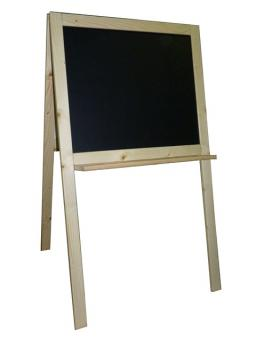We would be pleased if you find an error in the word problem, spelling mistakes, or inaccuracies and send it to us. Thank you!Tips to related online calculators
Would you like to compute count of combinations?

## Next similar math problems:

• Calculation of CNCalculate: ?
• TrinityHow many different triads can be selected from the group 43 students?
• ChordsHow many 4-tones chords (chord = at the same time sounding different tones) is possible to play within 7 tones?
• TeamsHow many ways can divide 16 players into two teams of 8 member?
• Fish tankA fish tank at a pet store has 8 zebra fish. In how many different ways can George choose 2 zebra fish to buy?
• DivisionDivision has 18 members: 10 girls and 6 boys, 2 leaders. How many different patrols can be created, if one patrol is 2 boys, 3 girls and 1 leader?
• BlocksThere are 9 interactive basic building blocks of an organization. How many two-blocks combinations are there?
• PIN - codesHow many five-digit PIN - code can we create using the even numbers?
• BallsThe urn is 8 white and 6 black balls. We pull 4 randomly balls. What is the probability that among them will be two white?
• VariationsDetermine the number of items when the count of variations of fourth class without repeating is 42 times larger than the count of variations of third class without repetition.
• ExaminationThe class is 21 students. How many ways can choose two to examination?On the menu are 12 kinds of meal. How many ways can we choose four different meals into the daily menu?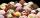The confectionery sold 5 kinds of ice cream. In how many ways can I buy 3 kinds if order of ice creams does not matter?A student is to answer 8 out of 10 questions on the exam. a) find the number n of ways the student can choose 8 out of 10 questions b) find n if the student must answer the first three questions c) How many if he must answer at least 4 of the first 5 ques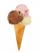Annie likes much ice cream. In the shop are six kinds of ice cream. In how many ways she can buy ice cream to three scoop if each have a different flavor mound and the order of scoops doesn't matter?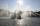The city has 7 fountains. Works only 6. How many options are there that can squirt ?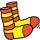In a class of 34 students, including 14 boys and 20 girls. How many couples (heterosexual, boy-girl) we can create? By what formula?